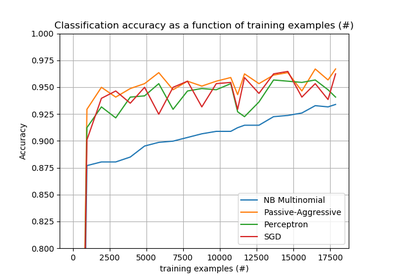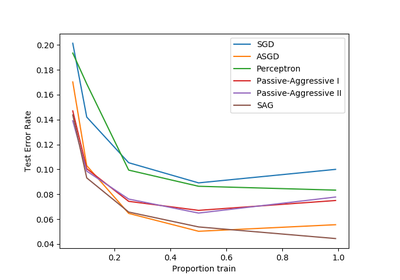# `sklearn.linear_model`.Perceptron¶

class `sklearn.linear_model.``Perceptron`(penalty=None, alpha=0.0001, fit_intercept=True, max_iter=None, tol=None, shuffle=True, verbose=0, eta0=1.0, n_jobs=1, random_state=0, class_weight=None, warm_start=False, n_iter=None)[source]

Read more in the User Guide.

Parameters: penalty : None, ‘l2’ or ‘l1’ or ‘elasticnet’ The penalty (aka regularization term) to be used. Defaults to None. alpha : float Constant that multiplies the regularization term if regularization is used. Defaults to 0.0001 fit_intercept : bool Whether the intercept should be estimated or not. If False, the data is assumed to be already centered. Defaults to True. max_iter : int, optional The maximum number of passes over the training data (aka epochs). It only impacts the behavior in the `fit` method, and not the partial_fit. Defaults to 5. Defaults to 1000 from 0.21, or if tol is not None. New in version 0.19. tol : float or None, optional The stopping criterion. If it is not None, the iterations will stop when (loss > previous_loss - tol). Defaults to None. Defaults to 1e-3 from 0.21. New in version 0.19. shuffle : bool, optional, default True Whether or not the training data should be shuffled after each epoch. verbose : integer, optional The verbosity level eta0 : double Constant by which the updates are multiplied. Defaults to 1. n_jobs : integer, optional The number of CPUs to use to do the OVA (One Versus All, for multi-class problems) computation. -1 means ‘all CPUs’. Defaults to 1. random_state : int, RandomState instance or None, optional, default None The seed of the pseudo random number generator to use when shuffling the data. If int, random_state is the seed used by the random number generator; If RandomState instance, random_state is the random number generator; If None, the random number generator is the RandomState instance used by np.random. class_weight : dict, {class_label: weight} or “balanced” or None, optional Preset for the class_weight fit parameter. Weights associated with classes. If not given, all classes are supposed to have weight one. The “balanced” mode uses the values of y to automatically adjust weights inversely proportional to class frequencies in the input data as `n_samples / (n_classes * np.bincount(y))` warm_start : bool, optional When set to True, reuse the solution of the previous call to fit as initialization, otherwise, just erase the previous solution. n_iter : int, optional The number of passes over the training data (aka epochs). Defaults to None. Deprecated, will be removed in 0.21. Changed in version 0.19: Deprecated coef_ : array, shape = [1, n_features] if n_classes == 2 else [n_classes, n_features] Weights assigned to the features. intercept_ : array, shape =  if n_classes == 2 else [n_classes] Constants in decision function. n_iter_ : int The actual number of iterations to reach the stopping criterion. For multiclass fits, it is the maximum over every binary fit.

Notes

Perceptron and SGDClassifier share the same underlying implementation. In fact, Perceptron() is equivalent to SGDClassifier(loss=”perceptron”, eta0=1, learning_rate=”constant”, penalty=None).

References

https://en.wikipedia.org/wiki/Perceptron and references therein.

Methods

 `decision_function`(X) Predict confidence scores for samples. `densify`() Convert coefficient matrix to dense array format. `fit`(X, y[, coef_init, intercept_init, …]) Fit linear model with Stochastic Gradient Descent. `get_params`([deep]) Get parameters for this estimator. `partial_fit`(X, y[, classes, sample_weight]) Fit linear model with Stochastic Gradient Descent. `predict`(X) Predict class labels for samples in X. `score`(X, y[, sample_weight]) Returns the mean accuracy on the given test data and labels. `set_params`(*args, **kwargs) `sparsify`() Convert coefficient matrix to sparse format.
`__init__`(penalty=None, alpha=0.0001, fit_intercept=True, max_iter=None, tol=None, shuffle=True, verbose=0, eta0=1.0, n_jobs=1, random_state=0, class_weight=None, warm_start=False, n_iter=None)[source]
`decision_function`(X)[source]

Predict confidence scores for samples.

The confidence score for a sample is the signed distance of that sample to the hyperplane.

Parameters: X : {array-like, sparse matrix}, shape = (n_samples, n_features) Samples. array, shape=(n_samples,) if n_classes == 2 else (n_samples, n_classes) : Confidence scores per (sample, class) combination. In the binary case, confidence score for self.classes_ where >0 means this class would be predicted.
`densify`()[source]

Convert coefficient matrix to dense array format.

Converts the `coef_` member (back) to a numpy.ndarray. This is the default format of `coef_` and is required for fitting, so calling this method is only required on models that have previously been sparsified; otherwise, it is a no-op.

Returns: self : estimator
`fit`(X, y, coef_init=None, intercept_init=None, sample_weight=None)[source]

Fit linear model with Stochastic Gradient Descent.

Parameters: X : {array-like, sparse matrix}, shape (n_samples, n_features) Training data y : numpy array, shape (n_samples,) Target values coef_init : array, shape (n_classes, n_features) The initial coefficients to warm-start the optimization. intercept_init : array, shape (n_classes,) The initial intercept to warm-start the optimization. sample_weight : array-like, shape (n_samples,), optional Weights applied to individual samples. If not provided, uniform weights are assumed. These weights will be multiplied with class_weight (passed through the constructor) if class_weight is specified self : returns an instance of self.
`get_params`(deep=True)[source]

Get parameters for this estimator.

Parameters: deep : boolean, optional If True, will return the parameters for this estimator and contained subobjects that are estimators. params : mapping of string to any Parameter names mapped to their values.
`loss_function`

DEPRECATED: Attribute loss_function was deprecated in version 0.19 and will be removed in 0.21. Use `loss_function_` instead

`partial_fit`(X, y, classes=None, sample_weight=None)[source]

Fit linear model with Stochastic Gradient Descent.

Parameters: X : {array-like, sparse matrix}, shape (n_samples, n_features) Subset of the training data y : numpy array, shape (n_samples,) Subset of the target values classes : array, shape (n_classes,) Classes across all calls to partial_fit. Can be obtained by via np.unique(y_all), where y_all is the target vector of the entire dataset. This argument is required for the first call to partial_fit and can be omitted in the subsequent calls. Note that y doesn’t need to contain all labels in classes. sample_weight : array-like, shape (n_samples,), optional Weights applied to individual samples. If not provided, uniform weights are assumed. self : returns an instance of self.
`predict`(X)[source]

Predict class labels for samples in X.

Parameters: X : {array-like, sparse matrix}, shape = [n_samples, n_features] Samples. C : array, shape = [n_samples] Predicted class label per sample.
`score`(X, y, sample_weight=None)[source]

Returns the mean accuracy on the given test data and labels.

In multi-label classification, this is the subset accuracy which is a harsh metric since you require for each sample that each label set be correctly predicted.

Parameters: X : array-like, shape = (n_samples, n_features) Test samples. y : array-like, shape = (n_samples) or (n_samples, n_outputs) True labels for X. sample_weight : array-like, shape = [n_samples], optional Sample weights. score : float Mean accuracy of self.predict(X) wrt. y.
`sparsify`()[source]

Convert coefficient matrix to sparse format.

Converts the `coef_` member to a scipy.sparse matrix, which for L1-regularized models can be much more memory- and storage-efficient than the usual numpy.ndarray representation.

The `intercept_` member is not converted.

Returns: self : estimator

Notes

For non-sparse models, i.e. when there are not many zeros in `coef_`, this may actually increase memory usage, so use this method with care. A rule of thumb is that the number of zero elements, which can be computed with `(coef_ == 0).sum()`, must be more than 50% for this to provide significant benefits.

After calling this method, further fitting with the partial_fit method (if any) will not work until you call densify.

## Examples using `sklearn.linear_model.Perceptron`¶Out-of-core classification of text documentsComparing various online solversClassification of text documents using sparse features